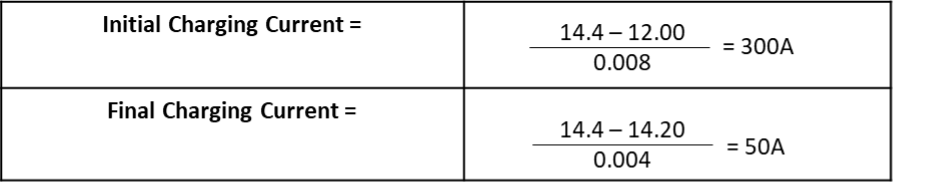# What is Constant Voltage (CV) charging?

Constant voltage (CV) allows the full current of the charger to flow into the battery until it reaches its pre-set voltage

CV is the preferred way of charging a battery in laboratories. However, a constant current (CC) charger with appropriate controls (referred to as charging algorithms or smart charging circuits) may also be used and, in fact, is the primary charger used in the OEM equipment market.

The following paragraphs are simplified for better understanding.

Basic electric circuit theory teaches us that the current in any circuit is directly proportional to the voltage potential or voltage differential between two points in a circuit (Ohm’s Law).

Ohm's law (ōmz) is a law that explains the voltage difference between two points, the electric current flowing between them, and the resistance of the path of the current. Mathematically, the law states that V = IR (Voltage = Current x Resistance) where V is the voltage difference, I is the current in amperes, and R is the resistance in ohms

If you know the total current and the voltage across the whole circuit at any snapshot in time, you can find the total resistance using Ohm's Law: R = V / I.

For example, a parallel circuit having a voltage of 12 volts and a total current of 3 amps is experiencing 4 Ohms of resistance. The total resistance RT = 12 volts / 3 amps = 4Ω. (4 Ohms)

Therefore, in theory, there is no limit on the charge current during a CV charge. However, many manufacturers publish limits as guidelines.

So as charging continues at a constant voltage, the charging current decreases due to the decreasing potential difference between the charger-output voltage and the battery terminal voltage as the battery charges. Expressed differently, the charging current is highest at the beginning of the charge cycle and lowest at the end of the charge cycle. Therefore, in a CV charge circuit, the battery is the current regulating device in the circuit. The battery will draw (or accept) only that amount of current as necessary to reach full charge. Once it attains a theoretical 100% state of charge, it will continue to draw small currents to compensate for standing/parasitic losses.

1. For example, let’s consider that the battery you are charging has an internal resistance of 8mΩ (0.008Ω) when fully discharged to an end voltage under a load of 1.75 volts per cell (10.5V for a 12V battery). And that it has an internal resistance of 4mΩ (0.004Ω) when fully charged.
2. We also know that the instant the load is removed from the battery during discharge that the voltage will jump back up to a higher voltage of around 2 volts per cell (12V for a 12V battery). This jump back is referred to as the back electromotive force (EMF) or counter electromotive force (CEMF). It is this voltage the charger will measure at the battery output terminals when the charging process begins. This voltage will influence the initial charge-current inrush and the final charging level.

Considering 1 and 2 above, we now decide to charge the battery using a constant voltage of 2.4 volts per cell (14.4V per battery). If we assume that the internal resistance of the battery when it is fully charged will be 4mΩ (0.004Ω), we can estimate what the finishing current will be when the battery is nearing a 100% state of chargeIn reality, the charging process is dynamic, so as soon as charging begins, the terminal voltage of a discharged battery begins rising to match the charger output voltage. Over time, one would expect the battery voltage at some point would equal the charger output voltage as the voltage difference in the charging circuit drops to theoretical zero. Thus, the charge current would drop to zero. However, this does not happen because of the internal electrochemistry, which ensures that the battery will keep drawing small charging currents even when fully charged.

The above example shows how the battery acts as a current regulator in a constant voltage charging regime, decreasing the current flow in the circuit to suit its state of charge. Thus, even if the current limit on the charger were 350 amperes, the battery would see an inrush current of 300 amperes before it tapered off and finally dropped to 50A towards the end of its charge.